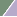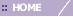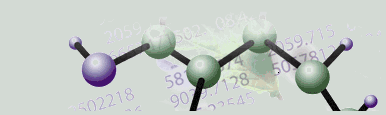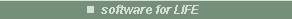Platinum

# Creating an ANN Classifier

Overview

In GeneLinker™, an ANN Classifier is actually a committee of artificial neural networks (ANNs).

Note: The terms Learner, Component Classifier, and Artificial Neural Network (ANN) are interchangeable. The term Classifier refers to an ensemble (committee) of learners.

Classifier Parameter Descriptions

Learners

• The number of learners to train. The samples are divided into N subsets. Each learner is trained on a different (N-1)/N samples and validated on the remaining 1/N samples. The default number is 10, corresponding to a conventional 10-fold cross-validation scheme. The number can be made as high as the number of samples (corresponding to leave-one-out cross-validation) or as low as 3. For most problems the default of 10 is fine.

• This is the number of learners which must vote for the same class in order for the Classifier to make a call (prediction) on a given sample. If fewer learners than this number agree, then the Classifier will make a class prediction of 'Unknown'. Raising this number may result in fewer misclassifications. Lowering it may lead to fewer 'Unknown' calls.

Hidden Units

• This is the number of nodes in the hidden layer of each ANN. All ANNs have the same three-layer architecture: input nodes , hidden nodes, and output nodes. You can think of each node as corresponding to a neuron, and the interconnections between them as synapses, but this model should not be taken too literally.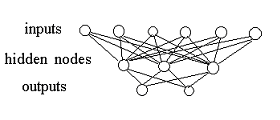• There are as many nodes in the input layer as there are input features (genes) in the training dataset. There are as many nodes in the output layer as there are output classes. The number of hidden nodes in the middle layer is typically between these two numbers.

• Setting the number of hidden nodes higher will usually result in overtraining, leading to poor results on test data. Setting the number of hidden nodes too low might result in an inability to learn even the training data, but this is easily detected by examining the results of the Create Classifier experiment. If the default number of hidden nodes yields good training results but poor test results, reduce the number of hidden nodes. If the default yields poor training results, try increasing the number of hidden nodes.

• Polak-Ribiere and Fletcher-Reeves are two variants of the conjugate gradient algorithm used to optimize the neural network internal parameters during training. They differ in the formula used to update the search direction in internal parameter space. For details see, for example, C.M. Bishop, 'Neural Networks for Pattern Recognition', Clarendon Press, Oxford, 1995.

Steps

• This is the number of conjugate gradient steps which the learner takes between evaluations of the stopping criteria.

Stopping Criteria: MSE Fractional Change

• Training of each ANN is stopped when the MSE (mean squared error) drops by less than this fraction between two successive iterations. The MSE is computed on the validation samples -- see 'Learners' above.

Stopping Criteria: Maximum Iterations

• The maximum number of times to evaluate the MSE for any individual ANN. An ANN may occasionally fail to reach the Stopping Criterion Threshold (above) even after running for a long time. This parameter limits the number of training cycles and prevents infinite loops.

Random Seed

• Randomization is used to select out the validation data for each learner, and to seed the internal parameters of each learner. Setting the random seed to a constant value is sometimes useful to determine exact sources of variation between different classifiers.

Actions

1. Click a dataset that has variable information associated with it in the Experiments navigator. The item is highlighted.

2. Click the Create ANN Classifier toolbar icon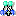, or select Create ANN Classifier from the Predict menu, or right-click the item and select Create ANN Classifier from the shortcut menu. The Create ANN Classifier parameters dialog is displayed.3. Set the parameters.

 Parameter Description Representative Variable A list of all the valid variables associated with this dataset are shown in the listbox. Select the variable that specifies the correct class values that the classifier is to be trained to predict. A "valid variable" is one that has all values for the variable represented in the dataset. For various reasons it is difficult to properly handle all cases where only a few of the possible values for a variable are represented in the training data. Learners The number of component learners in the classifier. Learner Votes Required The threshold at which the classifier will make a prediction. Hidden Units The number of nodes in the hidden layer of the learner. Conjugate Gradient Method Specifies the variant of the method to use. Steps The number of conjugate gradient steps between evaluations of the stopping criteria. MSE Fractional Change Learner training stops when the MSE drops less than this threshold between two successive iterations. Maximum Iterations The maximum number of times to evaluate the MSE for a learner. Random Seed Seed value for the random number generator.

4. Click OK. The Experiment Progress dialog is displayed. It is dynamically updated as the Create Classifier operation is performed. To cancel the Create Classifier operation, click the Cancel button.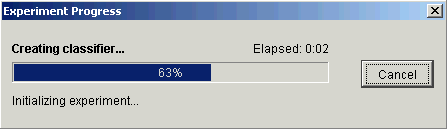Upon successful completion, a new item (Trained Classifier) is added under the original item in the Experiments navigator.

Related Topics:

ANN Classification and Prediction Overview

Classify New Data

Classification Plot

MSE Plot